# Geometry & Properties of Triangles Chapter Exam

Exam Instructions:

Choose your answers to the questions and click 'Next' to see the next set of questions. You can skip questions if you would like and come back to them later with the yellow "Go To First Skipped Question" button. When you have completed the practice exam, a green submit button will appear. Click it to see your results. Good luck!

### Page 2

#### Question 6 6. Find x.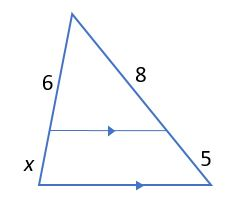#### Question 7 7. Find x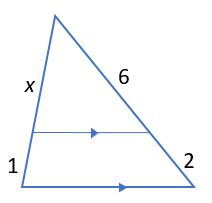#### Question 8 8. Find x.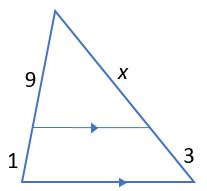### Page 4

#### Question 17 17. In the figure below, Angle 13 = 10y and Angle 11 = 3y + 11. Determine the measure of Angle 16.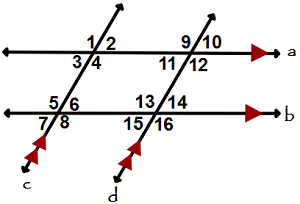#### Question 18 18. In triangle GBN, Angle G is seven more than three times a number, Angle B is five more than four times the number, and Angle N is twelve less than five times the number. Solve for the measure of Angle G.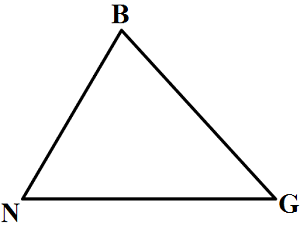#### Question 19 19. In the figure below, Angle 7 = 15x and Angle 10 = 9x + 30. Determine the measure of Angle 10.#### Question 20 20. Given the triangle ABC, solve for the value of y.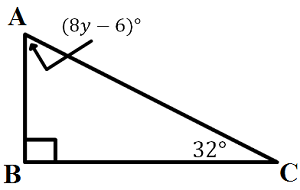### Page 5

#### Question 25 25. What type of triangle is pictured?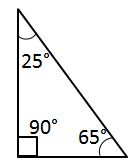### Page 6

#### Question 26 26. What type of triangle is pictured?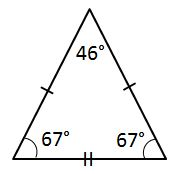#### Question 27 27. What type of triangle is pictured?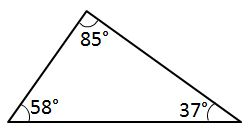#### Question 28 28. What type of triangle is pictured?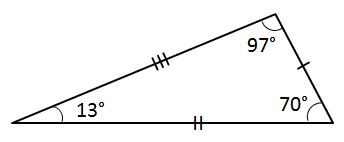#### Question 29 29. All angles that appear to be right angles are right angles. Can you tell from this picture that A = B?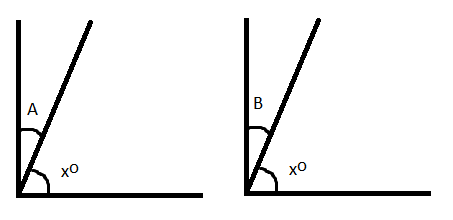#### Question 30 30. Find x.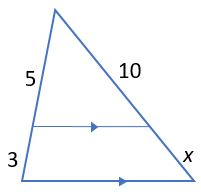#### Geometry & Properties of Triangles Chapter Exam Instructions

Choose your answers to the questions and click 'Next' to see the next set of questions. You can skip questions if you would like and come back to them later with the yellow "Go To First Skipped Question" button. When you have completed the practice exam, a green submit button will appear. Click it to see your results. Good luck!

Support